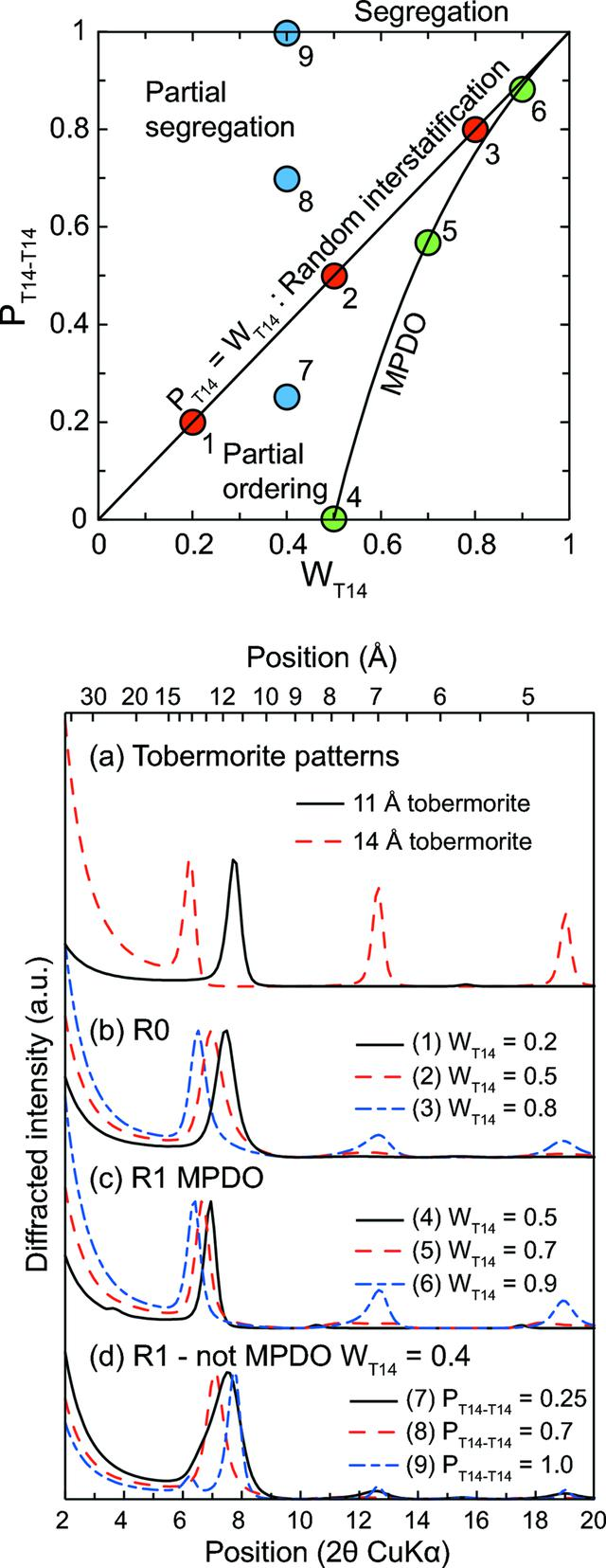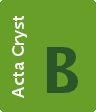disable zoom     view article Figure 6 Impact of interstratification on the 00l reflections from a theoretical mixed-layer tobermorite XRD pattern. (top) Different interstratification modes for a binary system containing tobermorite-like layers having 14 and 11 Å basal distances, plotted in a PT14-T14 versus WT14 graph. The structure of the 14 Å layer is that of plombierite, and the structure of the 11 Å layer is that from a MDO2 sample from Urals where all bridging tetrahedra are omitted. Solid line: field of R1 maximum possible degree of order (R1 MDPO), dotted line: field of R0 (random interstratification). Between these two lines lies the field of partial ordering, and above the R0 field lies the partial segregation field. Segregation is reached when PT14-T14 = 1. The area below the MPDO field is a non-physical field. Dots labelled 1–9 represent the nine calculations performed in the bottom figure: 1–3 are calculations performed in the R0 hypothesis (b in the bottom figure, with WT14 = 0.2, 0.5 and 0.8 for calculations 1 – solid line, 2 – dashed line and 3 – dash-dotted line), 4–6 calculations in the R1 MPDO hypothesis (c in the bottom figure, WT14 = 0.5, 0.7 and 0.9 for 4 – solid line, 5 – dashed line and 6 – dash–dotted line) and 7–9 calculations performed in the R1 non-MDPO hypothesis (d in the bottom figure, WT14 = 0.4 and PT14-T14 equal to 0.2, 0.5 and 1 for 7 – solid line, 8 – dashed line and 9 – dash–dotted line); (a) in the bottom figure presents the theoretical basal reflections from pure 11 and 14 Å tobermorite (solid and dashed lines, respectively).STRUCTURAL SCIENCECRYSTAL ENGINEERINGMATERIALS
ISSN: 2052-5206
Volume 69| Part 5| September 2013| Pages 465-473